# Discovery of the Neutron

It is remarkable that the neutron was not discovered until 1932 when James Chadwick used scattering data to calculate the mass of this neutral particle. Since the time of Rutherford it had been known that the atomic mass number A of nuclei is a bit more than twice the atomic number Z for most atoms and that essentially all the mass of the atom is concentrated in the relatively tiny nucleus. As of about 1930 it was presumed that the fundamental particles were protons and electrons, but that required that somehow a number of electrons were bound in the nucleus to partially cancel the charge of A protons. But by this time it was known from the uncertainty principle and from "particle-in-a-box" type confinement calculations that there just wasn't enough energy available to contain electrons in the nucleus.

A rough scale of the energy required for the confinement of a particle to a given dimension can be obtained by setting the DeBroglie wavelength of the particle equal to that dimension. For example, if we presume that the dimension of a hydrogen atom is about 0.2 nm, then the corresponding confinement energy is about 38 eV, the correct order of magnitude for atomic electrons. But to confine an electron to a nuclear dimension of about 5 fermis requires an energy of about 250 MeV. The maximum available confinement energy from the electrical attraction to the nucleus is given by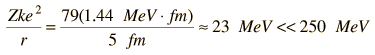Electrostaticpotential energy

So it is clear that there are no electrons in the nucleus.

An experimental breakthrough came in 1930 with the observation by Bothe and Becker that bombardment of beryllium with alpha particles from a radioactive source produced neutral radiation which was penetrating but non-ionizing. They presumed it was gamma rays, but Curie and Joliot showed that when you bombarded a paraffin target with this radiation, it ejected protons with energy about 5.3 MeV. This proved to be inconsistent with gamma rays, as can be shown from momentum and energy analysis: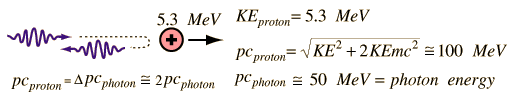This analysis follows that for a headon elastic collision where a small particle strikes a much more massive one. Again, the necessary energy for the gamma ray explanation was much greater than any energy observed to be available from the nucleus, so the neutral radiation must be some kind of neutral particle.

The 5.3 MeV energy of the ejected protons could be easily explained if the neutral particle had a mass comparable to that of the proton. For headon collisions, this would require only 5.3 MeV from the neutral particle, a value in the range of observed nuclear particle emissions.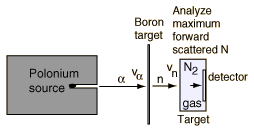Chadwick was able to prove that the neutral particle could not be a photon by bombarding targets other than hydrogen, including nitrogen, oxygen, helium and argon. Not only were these inconsistent with photon emission on energy grounds, the cross-section for the interactions was orders of magnitude greater than that for Compton scattering by photons.

The task which remained for Chadwick was that of determining the mass of the neutral particle. He chose to bombard boron with alpha particles and analyze the interaction of the neutral particles with nitrogen. These particlular targets were chosen partly because the masses of boron and nitrogen were well known. Conservation of energy applied to the combined interactions gives the following expressions: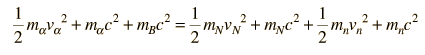Solving for the mass energy of the neutron gives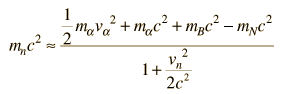The remaining unknown on the right hand side of the equation is the speed of the neutron. Assuming that the neutron mass was close to that of the proton, Chadwick bombarded hydrogen atoms with his produced neutrons to learn the speed of the protons after the collisions. Then setting the neutron speed equal to those proton speeds, he used the above energy expression to get a neutron mass of 938 +/- 1.8 MeV. With a consistent set of experiments, Chadwick had obtained the first value for the neutron mass which compared well with the presently accepted value of 939.57 MeV.

 The search for the basic structure of matter.
Index

Particle concepts

References
Rohlf
Ch. 11

Krane, Intro Nuclear Physics
Ch. 12

 HyperPhysics***** Quantum Physics R Nave
Go Back